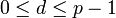# Modular representation theory of projective special linear group of degree two over a finite field in its defining characteristic

## Contents

This article gives specific information, namely, modular representation theory, about a family of groups, namely: projective special linear group of degree two.
View modular representation theory of group families | View other specific information about projective special linear group of degree two

This article discusses the modular representation theory in characteristic$p$ (where$p$ is a prime number) for the projective special linear group of degree two over a finite field of size$q$ and characteristic$p$. Note in particular that the characteristic of the field over which we are taking representations is the same as the characteristic of the field over which we are considering the projective special linear group of degree two.

## Particular cases$q$ (field size)$p$ (underlying prime, field characteristic) Group$PSL(2,q)$ Order of the group (=$(q^3 - q)/2$ if$q$ odd,$q^3 - q$ if$q$ even) GAP ID (second part) Degrees of irreducible representations in characteristic$p$ Number of irreducible representations (=$(q + 1)/2$ if$q$ odd,$q$ if$q$ even) Modular representation theory page
2 2 symmetric group:S3 6 1 1,2 2 modular representation theory of symmetric group:S3 at 2
3 3 special linear group:SL(2,3) 12 2 1,3 2 modular representation theory of alternating group:A4 at 3
4 2 alternating group:A5 60 5 1,2,2,4 4 modular representation theory of alternating group:A5 at 2
5 5 alternating group:A5 120 5 1,3,5 3 modular representation theory of alternating group:A5 at 5
7 7 projective special linear group:PSL(3,2) 168 42 1,3,5,7 4 modular representation theory of projective special linear group:PSL(3,2) at 7
8 2 projective special linear group:PSL(2,8) 504 156 1,2,2,2,4,4,4,8 8 modular representation theory of projective special linear group:PSL(2,8) at 2
9 3 alternating group:A6 360 118 1,3,3,4,9 5 modular representation theory of alternating group:A6 at 3

## Case$p = q$ is an odd prime

In this case, the degrees of irreducible representations are$1,3,5,\dots,p$ (a total of$(p + 1)/2$ representations) as follows:

• Consider the natural action of$SL(2,p)$ on a two-dimensional vector space over$\mathbb{F}_p$ with basis labeled$x,y$.
• Use this to induce an action of$SL(2,p)$ on the polynomial ring$\mathbb{F}_p[x,y]$.
• For$d$ a nonnegative integer, the space of homogeneous polynomials of degree$d$ is an invariant subspace of degree$d + 1$ with basis$x^d, x^{d-1}y, \dots, xy^{d-1},y^d$.$SL(2,p)$ thus acquires a representation on this space of degree$d + 1$. For the case$0 \le d \le p - 1$, the induced action on this space gives an irreducible representation of degree$d + 1$. When$d$ is even (so the degree$d + 1$ is odd) the center acts trivially, and we thus get a representation of the quotient group$PSL(2,p)$.

Note that for$d \ge p$, we still get a representation but this is not irreducible.

## General case

First, we recall the general case for the special linear group of degree two:

## General case

In this case, there are$q$ irreducible representations, given as follows. Suppose$q = p^r$ where$p$ is the characteristic.

Each irreducible representation can be described as a tensor product of$r$ representations:$\varphi_1 \otimes \varphi_2 \otimes \dots \otimes \varphi_r$

where each$\varphi_i$ is a representation of degree$d_i + 1$ obtained by looking at homogeneous degree$d_i$ polynomials in$\mathbb{F}_q[x,y]$ and where the action is given by composing the usual$SL_2$-action with the$i^{th}$ power of Frobenius, i.e., with the field automorphism$t \mapsto t^{p^i}$.

As before, we have$0 \le d_i \le p - 1$, so we get the following degree for the whole representation:$\prod_{i=1}^r (d_i + 1)$

This number is bounded from below by$1$ and from above by$q$. However, for$r > 1$, it is not the case that each degree appears once. Rather, each degree appears based on the number of distinct ways it can be written as an ordered product of numbers between 1 and$p$.

For$p = 2$, all these descend to the projective special linear group because the special linear group is the same as the projective special linear group.

Of these$q$ representations, not all descend to the projective special linear group in the case of odd$q$. For odd$q$, the criterion for a representation to descend is the following: The number of$d_i$s that are even (so that$d_i + 1$ is odd) should be even.

A short combinatorial argument shows that there are exactly$(q + 1)/2$ possible ways of choosing these$d_i$s when$q$ is odd.